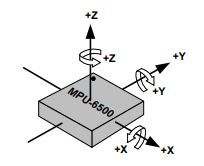# QQuaternion rotation of accelerometer data

• Hi All,

I need to rotate the accelerometer data from the sensor coordinate frame to the world coordinate frame.

I did the following Quaternion multiplication (`v' = qvq*`), where `v'` is the rotated accelerometer vector.

My quaternion are in the form of (`q0, q1, q2, q3`), where `q0` is the scalar component.

``````QQuaternion q;
q.setScalar(q0);
q.setX(q1);
q.setY(q2);
q.setZ(q3);

QVector3D aVector(ax,ay,az); // acceleration vector
QVector3D qRotated = q.rotatedVector(vector); // this is v'
``````

However, this does not seems working, I have difficulty in understanding if it's due to the my quaternion values or the way I'm dealing with the rotation. Does anybody have some idea?

• I have difficulty in understanding if it's due to the my quaternion values

Probably. How are these numbers obtained? Can you provide an example of the actual numbers as well?

• That's generated by the IMU sensor, I then normalized the quaternion as follows:

`````` double q_mag=sqrt(q0*q0 + q1*q1 + q2*q2 + q3*q3);
q0/=q_mag;
q1/=q_mag;
q2/=q_mag;
q3/=q_mag;
``````

I tried to visualize the quaternion rotation, using a cube model, which looks ok. A typical value of quaternion is below:

``````q0 =  0.450496
q1 = -0.00253865
q2 = -0.0104597
q3 = -0.892714
``````

• What are you trying to do exactly, it's not that clear from the description. The quaternion you get has what meaning with relation to the vector? Is the vector sampled directly and the quaternion is the sensor's orientation? That's how I read the question. If so then you invert the quaternion and apply it to the vector to get the latter in the global reference (i.e. the one that the sensor's in).
Inverting a quaternion is trivial - conjugating and normalizing it (to the square length) it just entails flipping the imaginary components' signs.

I did the following Quaternion multiplication (v' = qvq*), where v' is the rotated accelerometer vector.

When, how? Is the `v'` the unknown or the known?

• @viniltc Of course, you need to very careful that you have the definitions correct for the sensor and world coordinate frames. For example, is the world frame Earth-centred rotating, or is it x-east, y-north, z-upward pointing, at the point below the sensor? Is the sensor x-forward, y-starboard, z-down? There are many variations of this that vendors can use, and documentation is often skimpy.

• What I'm aiming to do is to compensate gravity from the accelerometer data. The acceleration vector (`QVector3D aVector(ax,ay,az)`) is sampled directly from the sensor.

What I then need to do is to transfer from local sensor from to the world frame using quaternion.

So I did this:

``````QQuaternion q; // quaternion
q.setScalar(q0);
q.setX(q1);
q.setY(q2);
q.setZ(q3);

QVector3D aVector(ax,ay,az); // acceleration vector
QVector3D qRotated = q.rotatedVector(vector); // convert acceleration from local frame to world frame
``````

then the next step is to subtract the gravity component from acceleration

``````QVector3D g(0,0,1);
QVector3D result = qRotated-g;
``````

Inverting a quaternion is trivial - conjugating and normalizing it (to the square length) it just entails flipping the imaginary components' signs.

So you suggest to invert quaternion first and rotate the acceleration, something like following?

``````QVector3D qRotated = q.inverted().rotatedVector(vector);
``````

• @normvcr thanks for your feedback.
I have a basic understanding of IMU. I was in an assumption that the world frame always the same (East, North, Up) .

My requirement is to use IMU as a shoulder sensor. My confusion is, how the quaternion rotation will affect based on the different conventions of world frame?

I can confirm my IMU frame looks like this from the datasheet:• I can confirm my IMU frame looks like this from the datasheet

Okay this gives enough info about the vector you get. It's sampled in that coordinate system. I still have no idea what the quaternion is supposed to represent. If it's the orientation of the sensor in relation to the fixed reference frame (i.e. "world"), then just inverting the quaternion and applying it to the vector should be sufficient. Did you try that?

So you suggest to invert quaternion first and rotate the acceleration, something like following?

Yes.

• @kshegunov , @normvcr Thanks a lot for your feedback. This got solved. It was too late to realise that the sign of the quaternion values was wrong in my case :)